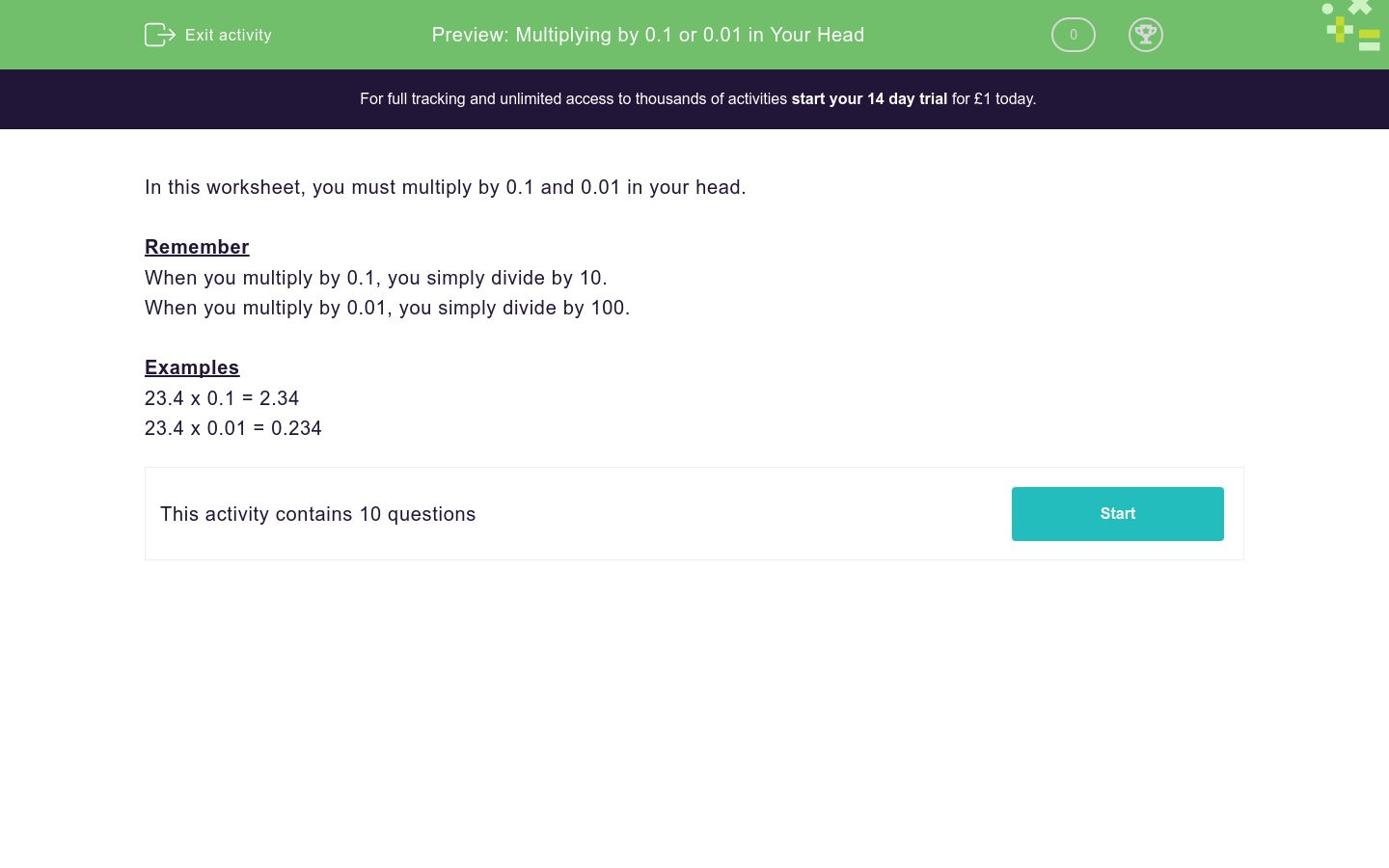### Affordable pricing from £10/month

In this worksheet, students multiply the given numbers by 0.1 or 0.01 in their heads.Key stage:  KS 3

Curriculum topic:   Number

Curriculum subtopic:   Use Four Operations for All Numbers

Difficulty level:### QUESTION 1 of 10

In this worksheet, you must multiply by 0.1 and 0.01 in your head.

Remember

When you multiply by 0.1, you simply divide by 10.

When you multiply by 0.01, you simply divide by 100.

Examples

23.4 x 0.1 = 2.34

23.4 x 0.01 = 0.234

35 × 0.1 = _______

198 × 0.01 = _______

426 × 0.1 = _______

83 × 0.01 = _______

439 × 0.1 = _______

116 × 0.1 = _______

444 × 0.1 = _______

675 × 0.1 = _______

967 × 0.1 = _______

3.29 × 0.01 = _______

• Question 1

35 × 0.1 = _______

3.5
• Question 2

198 × 0.01 = _______

1.98
• Question 3

426 × 0.1 = _______

42.6
• Question 4

83 × 0.01 = _______

0.83
• Question 5

439 × 0.1 = _______

43.9
• Question 6

116 × 0.1 = _______

11.6
• Question 7

444 × 0.1 = _______

44.4
• Question 8

675 × 0.1 = _______

67.5
• Question 9

967 × 0.1 = _______

96.7
• Question 10

3.29 × 0.01 = _______

0.0329
---- OR ----

Sign up for a £1 trial so you can track and measure your child's progress on this activity.

### What is EdPlace?

We're your National Curriculum aligned online education content provider helping each child succeed in English, maths and science from year 1 to GCSE. With an EdPlace account you’ll be able to track and measure progress, helping each child achieve their best. We build confidence and attainment by personalising each child’s learning at a level that suits them.

Get started